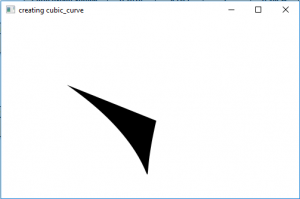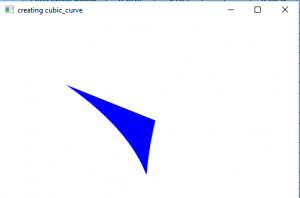# JavaFX | CubicCurve with examples

CubicCurve is a part of JavaFX.The CubiCurve class defines a cubic Bézier parametric curve segment in (x, y) coordinate space. The Cubic curve passes through the starting point and the ending point and also passes through the two control points. The control points are specified as Bézier control points.

Constructor for the class are :

1. CubicCurve(): creates a new instance of cubic curve
2. CubicCurve(double startX, double startY, double controlX1, double controlY1, double controlX2, double controlY2, double endX, double endY) : creates a instance of cubic curve with specified starting and ending points and two control points.

commonly used methods

Method Explanation
getControlX1() returns the x coordinate of 1st control point
getControlY1() returns the y coordinate of 1st control point
getControlX2() returns the x coordinate of 2nd control point
getControlY2() returns the y coordinate of 2nd control point
getEndX() returns the x coordinate for the end point
getEndY() returns the y coordinate for the end point
getStartX() returns the x coordinate for the start point
getStartY() returns the y coordinate for the start point
setControlX1(double value) sets the x coordinate for the 1st control point
setControlY1(double value) sets the y coordinate for the 1st control point
setControlX2(double value) sets the x coordinate for the 2nd control point
setControlY2(double value) sets the y coordinate for the 2nd control point
setEndX(double value) sets the x coordinate for the end point
setEndY(double value) sets the y coordinate for the end point
setStartX(double value) sets the x coordinate for the start point
setStartY(double value) sets the y coordinate for the start point

Java program to create a cubic curve

This program creates a CubicCurve indicated by the name cubic_curve( two control point s, start point and end point is passed as arguments). The CubicCurve will be created inside a scene, which in turn will be hosted inside a stage. The function setTitle() is used to provide title to the stage. Then a Group is created, and the cubic_curve is attached.The group is attached to the scene. Finally, the show() method is called to display the final results.

 `// Java program to create a cubic curve ` `import` `javafx.application.Application; ` `import` `javafx.scene.Scene; ` `import` `javafx.scene.shape.DrawMode; ` `import` `javafx.scene.layout.*; ` `import` `javafx.event.ActionEvent; ` `import` `javafx.scene.shape.CubicCurve; ` `import` `javafx.scene.control.*; ` `import` `javafx.stage.Stage; ` `import` `javafx.scene.Group; ` `public` `class` `cubic_curve_0 ``extends` `Application { ` ` `  `    ``// launch the application ` `    ``public` `void` `start(Stage stage) ` `    ``{ ` `        ``// set title for the stage ` `        ``stage.setTitle(``"creating cubic_curve"``); ` ` `  `        ``// create a cubic_curve ` `        ``CubicCurve cubic_curve = ``new` `CubicCurve(``10``.0f, ``10``.0f, ``200``.0f, ``140``.0f, ``120``.0f, ``240``.0f, ``160``.0f, ``70``.0f); ` ` `  `        ``// create a Group ` `        ``Group group = ``new` `Group(cubic_curve); ` ` `  `        ``// translate the cubic_curve to a position ` `        ``cubic_curve.setTranslateX(``100``); ` `        ``cubic_curve.setTranslateY(``100``); ` ` `  `        ``// create a scene ` `        ``Scene scene = ``new` `Scene(group, ``500``, ``300``); ` ` `  `        ``// set the scene ` `        ``stage.setScene(scene); ` ` `  `        ``stage.show(); ` `    ``} ` ` `  `    ``public` `static` `void` `main(String args[]) ` `    ``{ ` `        ``// launch the application ` `        ``launch(args); ` `    ``} ` `} `

Output:Java program to create a cubic curve and set a fill for cubic curve

This program creates a CubicCurve indicated by the name cubic_curve(two control points, start point and end point are set using setControlX1(), setControlY1(), setControlX2(), setControlY2(), setStartX(), setStartY(), setEndX(), ans setEndY() functions). The CubicCurvewill be created inside a scene, which in turn will be hosted inside a stage. The function setTitle() is used to provide title to the stage. Then a Group is created, and the cubic_curve is attached.The group is attached to the scene. Finally, the show() method is called to display the final results.The function setFill() is used to set fill for the cubic curve.

 `// Java program to create a cubic curve and set a fill for cubic curve ` `import` `javafx.application.Application; ` `import` `javafx.scene.Scene; ` `import` `javafx.scene.shape.DrawMode; ` `import` `javafx.scene.layout.*; ` `import` `javafx.event.ActionEvent; ` `import` `javafx.scene.shape.CubicCurve; ` `import` `javafx.scene.control.*; ` `import` `javafx.stage.Stage; ` `import` `javafx.scene.Group; ` `import` `javafx.scene.paint.Color; ` `public` `class` `cubic_curve_1 ``extends` `Application { ` ` `  `    ``// launch the application ` `    ``public` `void` `start(Stage stage) ` `    ``{ ` `        ``// set title for the stage ` `        ``stage.setTitle(``"creating cubic_curve"``); ` ` `  `        ``// create a cubic_curve ` `        ``CubicCurve cubic_curve = ``new` `CubicCurve(); ` ` `  `        ``// set start ` `        ``cubic_curve.setStartX(``10``.0f); ` `        ``cubic_curve.setStartY(``10``.0f); ` ` `  `        ``// set control coordinates ` `        ``cubic_curve.setControlX1(``200``.0f); ` `        ``cubic_curve.setControlY1(``140``.0f); ` `        ``cubic_curve.setControlX2(``120``.0f); ` `        ``cubic_curve.setControlY2(``240``.0f); ` ` `  `        ``// set end coordinates ` `        ``cubic_curve.setEndX(``160``.0f); ` `        ``cubic_curve.setEndY(``70``.0f); ` ` `  `        ``// create a Group ` `        ``Group group = ``new` `Group(cubic_curve); ` ` `  `        ``// translate the cubic_curve to a position ` `        ``cubic_curve.setTranslateX(``100``); ` `        ``cubic_curve.setTranslateY(``100``); ` ` `  `        ``// set fill for the cubic curve ` `        ``cubic_curve.setFill(Color.BLUE); ` ` `  `        ``// create a scene ` `        ``Scene scene = ``new` `Scene(group, ``500``, ``300``); ` ` `  `        ``// set the scene ` `        ``stage.setScene(scene); ` ` `  `        ``stage.show(); ` `    ``} ` ` `  `    ``public` `static` `void` `main(String args[]) ` `    ``{ ` `        ``// launch the application ` `        ``launch(args); ` `    ``} ` `} `

Output:Note : The above programs might not run in online IDE please use an offline compiler
Reference:
https://docs.oracle.com/javase/8/javafx/api/javafx/scene/shape/CubicCurve.html

My Personal Notes arrow_drop_upCheck out this Author's contributed articles.

If you like GeeksforGeeks and would like to contribute, you can also write an article using contribute.geeksforgeeks.org or mail your article to contribute@geeksforgeeks.org. See your article appearing on the GeeksforGeeks main page and help other Geeks.

Please Improve this article if you find anything incorrect by clicking on the "Improve Article" button below.

Article Tags :

Be the First to upvote.

Please write to us at contribute@geeksforgeeks.org to report any issue with the above content.